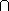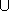## CGAL::Nef_polyhedron_2<T>

### Definition

An instance of data type Nef_polyhedron_2<T> is a subset of the plane that is the result of forming complements and intersections starting from a finite set H of halfspaces. Nef_polyhedron_2 is closed under all binary set operations intersection, union, difference, complement and under the topological operations boundary, closure, and interior.

The template parameter T is specified via an extended kernel concept. T must be a model of the concept ExtendedKernelTraits_2.

#include <CGAL/Nef_polyhedron_2.h>

### Types

 Nef_polyhedron_2::Line the oriented lines modeling halfplanes Nef_polyhedron_2::Point the affine points of the plane. Nef_polyhedron_2::Direction directions in our plane. enum Boundary { EXCLUDED, INCLUDED}; construction selection. enum Content { EMPTY, COMPLETE}; construction selection

### Creation

 Nef_polyhedron_2 N ( Content plane = EMPTY); creates an instance N of type Nef_polyhedron_2 and initializes it to the empty set if plane == EMPTY and to the whole plane if plane == COMPLETE. Nef_polyhedron_2 N ( Line l, Boundary line = INCLUDED); creates a Nef polyhedron N containing the halfplane left of l including l if line==INCLUDED, excluding l if line==EXCLUDED. template Nef_polyhedron_2 N ( Forward_iterator it, Forward_iterator end, Boundary b = INCLUDED); creates a Nef polyhedron N from the simple polygon P spanned by the list of points in the iterator range [it,end) and including its boundary if b = INCLUDED excluding the boundary otherwise. Forward_iterator has to be an iterator with value type Point. This construction expects that P is simple. The degenerate cases where P contains no point, one point or spans just one segment (two points) are correctly handled. In all degenerate cases there's only one unbounded face adjacent to the degenerate polygon. If b == INCLUDED then N is just the boundary. If b == EXCLUDED then N is the whole plane without the boundary.

### Operations

 void N.clear ( Content plane = EMPTY) makes N the empty set if plane == EMPTY and the full plane if plane == COMPLETE. bool N.is_empty () returns true if N is empty, false otherwise. bool N.is_plane () returns true if N is the whole plane, false otherwise.

### Constructive Operations

 Nef_polyhedron_2 N.complement () returns the complement of N in the plane. Nef_polyhedron_2 N.interior () returns the interior of N. Nef_polyhedron_2 N.closure () returns the closure of N. Nef_polyhedron_2 N.boundary () returns the boundary of N. Nef_polyhedron_2 N.regularization () returns the regularized polyhedron (closure of interior). Nef_polyhedron_2 N.intersection ( N1) returns NN1. Nef_polyhedron_2 N.join ( N1) returns NN1. Note that ``union'' is a keyword of C++ and cannot be used for this operation. Nef_polyhedron_2 N.difference ( N1) returns N - N1. Nef_polyhedron_2 N.symmetric_difference ( N1) returns the symmectric difference N - TT - N.

Additionally there are operators *,+,-,^,! which implement the binary operations intersection, join, difference, symmetric difference, and the unary operation complement, respectively. There are also the corresponding modification operations *=,+=,-=,^=.

There are also comparison operations like <,<=,>,>=,==,!= which implement the relations subset, subset or equal, superset, superset or equal, equality, inequality, respectively.

### Exploration - Point location - Ray shooting

As Nef polyhedra are the result of forming complements and intersections starting from a set H of halfspaces that are defined by oriented lines in the plane, they can be represented by an attributed plane map M = (V,E,F). For topological queries within M the following types and operations allow exploration access to this structure.

### Types

 Nef_polyhedron_2::Explorer a decorator to examine the underlying plane map. See the manual page of Explorer. Nef_polyhedron_2::Object_handle a generic handle to an object of the underlying plane map. The kind of object (vertex, halfedge, face) can be determined and the object can be assigned to a corresponding handle by the three functions: bool assign(Vertex_const_handle& h, Object_handle) bool assign(Halfedge_const_handle& h, Object_handle) bool assign(Face_const_handle& h, Object_handle) where each function returns true iff the assignment to h was done. enum Location_mode { DEFAULT, NAIVE, LMWT}; selection flagfor the point location mode. LMWT stands for Locally Minimum Weight Triangulation, a locally optimized constrained triangulation where the weight corresponds to the lenght of the edges of the triangulation.

### Operations

 bool N.contains ( Object_handle h) returns true iff the object h is contained in the set represented by N. bool N.contained_in_boundary ( Object_handle h) returns true iff the object h is contained in the 1-skeleton of N. Object_handle N.locate ( Point p, Location_mode m = DEFAULT) returns a generic handle h to an object (face, halfedge, vertex) of the underlying plane map that contains the point p in its relative interior. The point p is contained in the set represented by N if N.contains(h) is true. The location mode flag m allows one to choose between different point location strategies. Object_handle N.ray_shoot ( Point p, Direction d, Location_mode m = DEFAULT) returns a handle h with N.contains(h), that can be converted to a Vertex_/Halfedge_/Face_const_handle as described above. The object returned is intersected by the ray starting in p with direction d and has minimal distance to p. The operation returns the null handle NULL if the ray shoot along d does not hit any object h of N with N.contains(h). The location mode flag m allows one to choose between different point location strategies. Object_handle N.ray_shoot_to_boundary ( Point p, Direction d, Location_mode m = DEFAULT) returns a handle h, that can be converted to a Vertex_/Halfedge_const_handle as described above. The object returned is part of the 1-skeleton of N, intersected by the ray starting in p with direction d and has minimal distance to p. The operation returns the null handle NULL if the ray shoot along d does not hit any 1-skeleton object h of N. The location mode flag m allows one to choose between different point location strategies. Explorer N.explorer () returns a decorator object that allows read-only access of the underlying plane map. See the manual page Explorer for its usage.

### Input and Output

A Nef polyhedron N can be visualized in a Window_stream W. The output operator is defined in the file CGAL/IO/Nef_-poly-hedron_2_-Win-dow_-stream.h.

### Implementation

Nef polyhedra are implemented on top of a halfedge data structure and use linear space in the number of vertices, edges and facets. Operations like empty take constant time. The operations clear, complement, interior, closure, boundary, regularization, input and output take linear time. All binary set operations and comparison operations take time O(n logn) where n is the size of the output plus the size of the input.

The point location and ray shooting operations are implemented in two flavors. The NAIVE operations run in linear query time without any preprocessing, the DEFAULT operations (equals LMWT) run in sub-linear query time, but preprocessing is triggered with the first operation. Preprocessing takes time O(N2), the sub-linear point location time is either logarithmic when LEDA's persistent dictionaries are present or if not then the point location time is worst-case linear, but experiments show often sublinear runtimes. Ray shooting equals point location plus a walk in the constrained triangulation overlayed on the plane map representation. The cost of the walk is proportional to the number of triangles passed in direction d until an obstacle is met. In a minimum weight triangulation of the obstacles (the plane map representing the polyhedron) the theory provides a O(sqrt(n)) bound for the number of steps. Our locally minimum weight triangulation approximates the minimum weight triangulation only heuristically (the calculation of the minimum weight triangulation is conjectured to be NP hard). Thus we have no runtime guarantee but a strong experimental motivation for its approximation.

### Example

Nef polyhedra are parameterized by a so-called extended geometric kernel. There are three kernels, one based on a homogeneous representation of extended points called Extended_homogeneous<RT> where RT is a ring type providing additionally a gcd operation, one based on a Cartesian representation of extended points called Extended_cartesian<NT> where NT is a field type, and finally Filtered_extended_homogeneous<RT> (an optimized version of the first). The following example uses the filtered homogeneous kernel to construct the intersection of two halfspaces.

```File: examples/Nef_2/simple_intersection.cpp
```
```#include <CGAL/Gmpz.h>
#include <CGAL/Filtered_extended_homogeneous.h>
#include <CGAL/Nef_polyhedron_2.h>

typedef CGAL::Gmpz RT;
typedef CGAL::Filtered_extended_homogeneous<RT> Extended_kernel;
typedef CGAL::Nef_polyhedron_2<Extended_kernel> Nef_polyhedron;
typedef Nef_polyhedron::Line  Line;

int main()
{
Nef_polyhedron N1(Line(1,0,0));
Nef_polyhedron N2(Line(0,1,0), Nef_polyhedron::EXCLUDED);
Nef_polyhedron N3 = N1 * N2; // line (*)
return 0;
}
```

After line (*) N3 is the intersection of N1 and N2. The member types of Nef_polyhedron_2< Extended_homogeneous<NT> > map to corresponding types of the standard CGAL geometry kernel (type equality in pseudo-code notation):

```CGAL::Nef_polyhedron_2< CGAL::Extended_cartesian<FT> >::Point
== CGAL::Cartesian<FT>::Point_2

CGAL::Nef_polyhedron_2< CGAL::Extended_homogeneous<RT> >::Point
== CGAL::Homogeneous<RT>::Point_2

CGAL::Nef_polyhedron_2< CGAL::Filtered_extended_homogeneous<RT> >::Point
== CGAL::Homogeneous<RT>::Point_2
```
The same holds for the types Line and Direction in the local scope of Nef_polyhedron_2<...>.